# Samacheer Kalvi 11th Maths Solutions Chapter 8 Vector Algebra – I Ex 8.2

## Tamilnadu Samacheer Kalvi 11th Maths Solutions Chapter 8 Vector Algebra – I Ex 8.2

Question 1.
Verify whether the following ratios are direction cosines of some vector or not.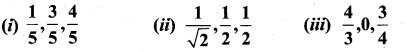Solution: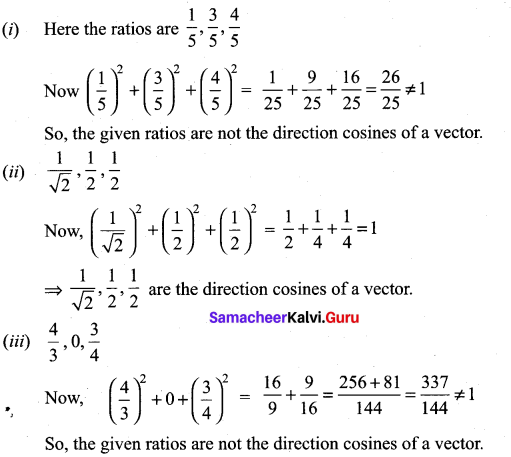Question 2.
Find the direction cosines of a vectors whose direction ratios are
(i) 1, 2, 3
(ii) 3, -1, 3
(iii) 0, 0, 7
Solution: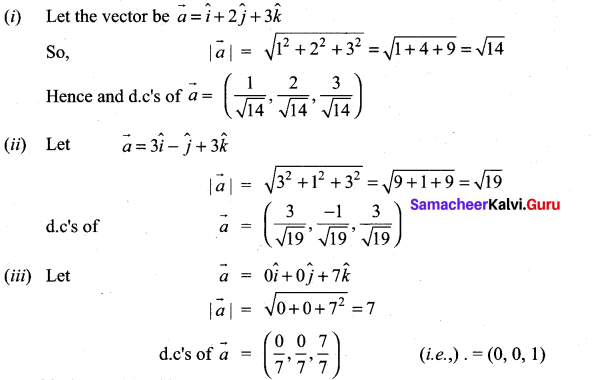Question 3.
Find the direction cosines and direction ratios for the following vectors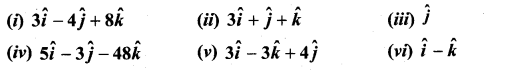Solution: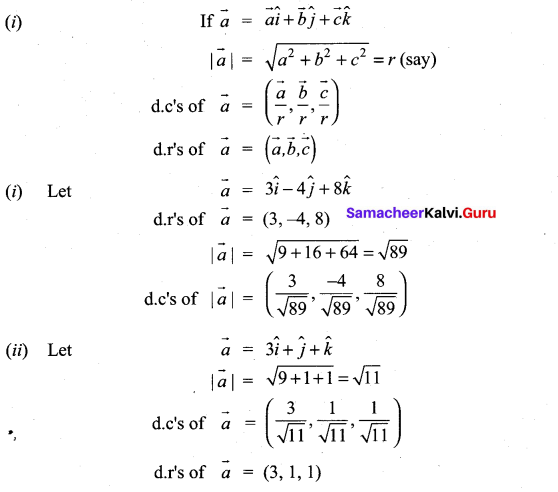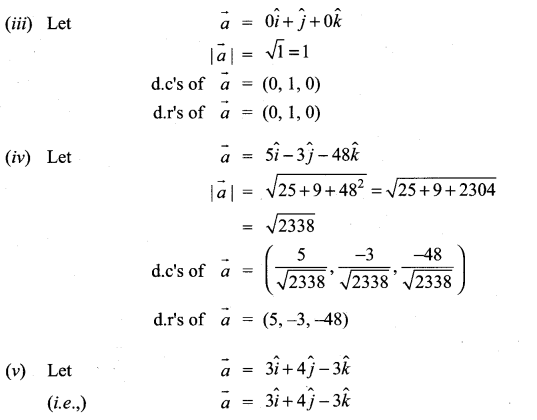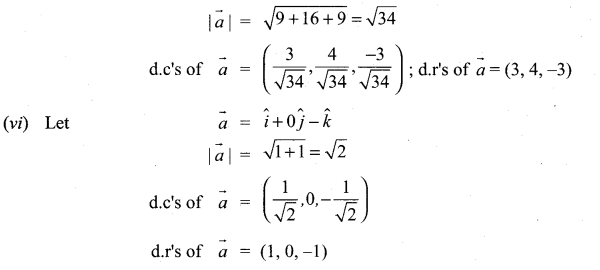Question 4.
A triangle is formed by joining the points (1, 0, 0), (0, 1, 0) and (0, 0, 1). Find the direction cosines of the medians.
Solution: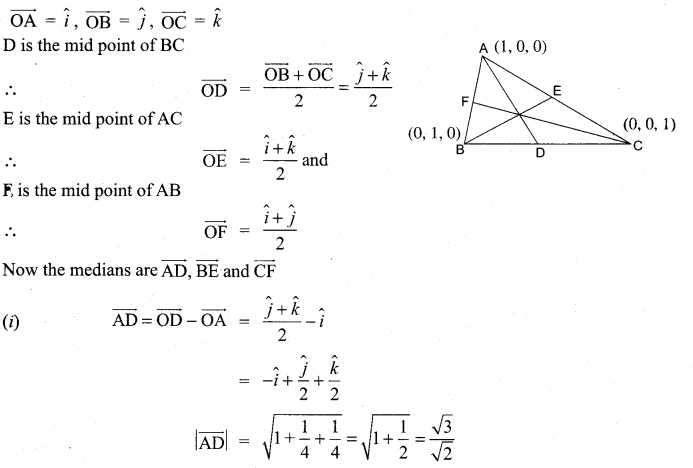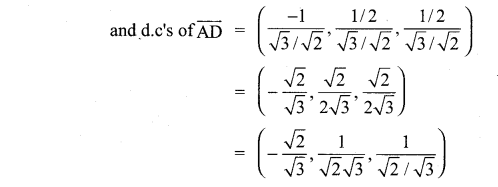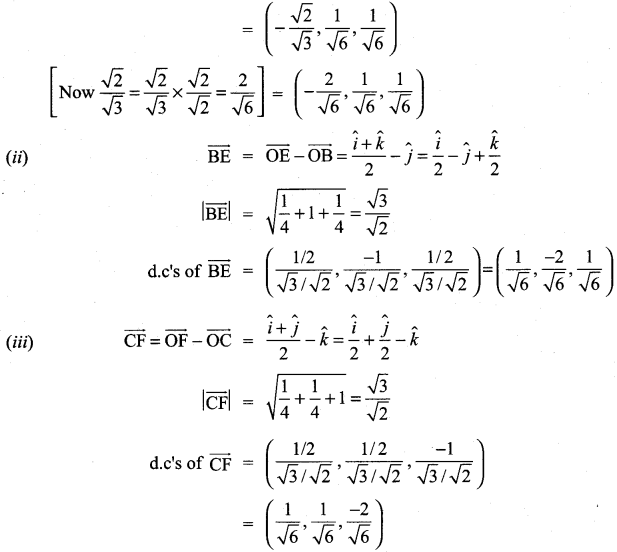Question 5.
If $$\frac{1}{2}, \frac{1}{\sqrt{2}}$$, a are the direction cosines of some vector, then find a.
Solution: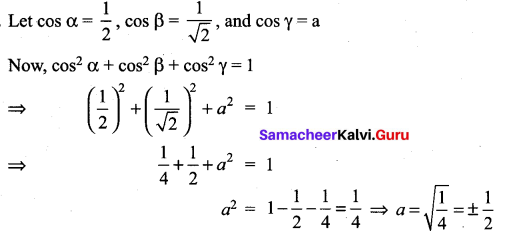Question 6.
If (a, a + b, a + b + c) is one set of direction ratios of the line joining (1, 0, 0) and (0, 1, 0), then find a set of values of a, b, c.
Solution:
Let A be the point (1, 0, 0) and B be the point (0, 1, 0) (i.e.,) $$\overrightarrow{\mathrm{OA}}=\hat{i}$$ and $$\overrightarrow{\mathrm{OB}}=\hat{j}$$
Then $$\overrightarrow{\mathrm{AB}}=\overrightarrow{\mathrm{OB}}-\overrightarrow{\mathrm{OA}}=\hat{j}-\hat{i}=-\hat{i}+\hat{j}$$
= (-1, 1, 0)
= (a, a + b, a + b + c)
⇒ a = -1, a + b = 1 and a + b + c = 0
Now a = -1 ⇒ -1 + b = 1 ;a + b + c = 0
⇒ b = 2; -1 + 2 + c = 0 ⇒ c + 1 = 0
⇒ c = -1
∴ a = -1; b = 2; c = -1.
Note: If we taken $$\overrightarrow{\mathrm{BA}}$$ then we get a = 1, b = -2 and c = 1.

Question 7.
Show that the vectors $$2 \hat{i}-\hat{j}+\hat{k}, 3 \hat{i}-4 \hat{j}-4 \hat{k}, \hat{i}-3 \hat{j}-5 \hat{k}$$ form a right angled triangle.
Sol: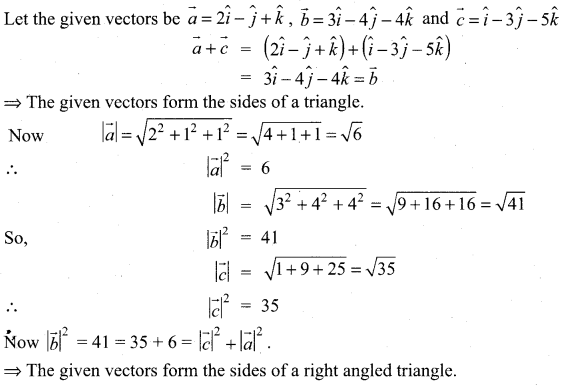⇒ The given vectors form the sides of a right angled triangle.Question 8.
Find the value of k for which the vectors $$\vec{a}=3 \hat{i}+2 \hat{j}+9 \hat{k}$$ and $$\vec{b}=\hat{i}+\lambda \hat{j}+3 \hat{k}$$ are parallel.
Solution: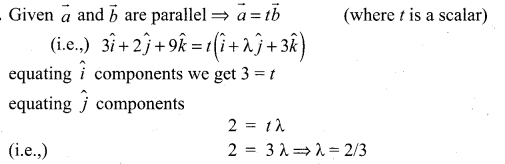Question 9.
Show that the following vectors are coplanar.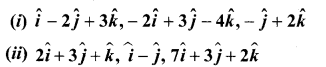Solution:
Let the given three vectors be $$\vec{a}$$, $$\vec{b}$$ and $$\vec{c}$$. When we are able to write one vector as a linear combination of the other two vectors, then the given vectors are called coplanar vectors.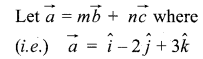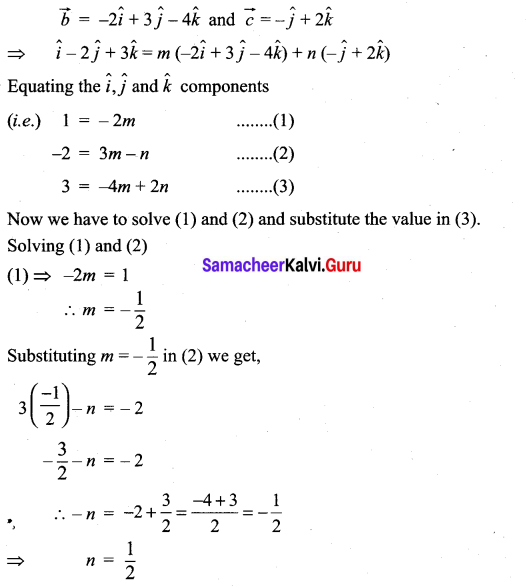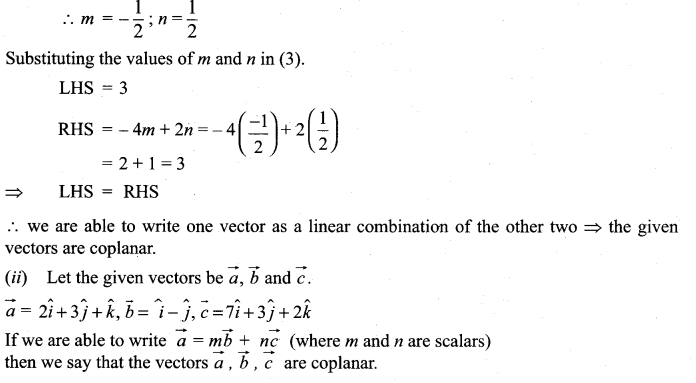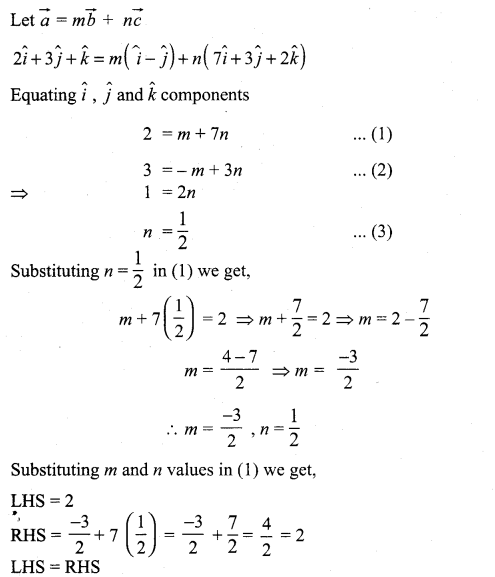We are able to write $$\vec{a}$$ as a linear combination of $$\vec{b}$$ and $$\vec{c}$$
∴ The vectors $$\vec{a}$$, $$\vec{b}$$, $$\vec{c}$$ are coplanar

Question 10.
Show that the points whose position vectors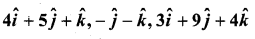and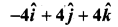are coplanar
Solution:
Let the given points be A, B, C and D. To prove that the points A, B, C, D are coplanar, we have to prove that the vectors $$\overrightarrow{\mathrm{AB}}, \overrightarrow{\mathrm{AC}}$$ and $$\overrightarrow{\mathrm{AC}}$$ are coplanar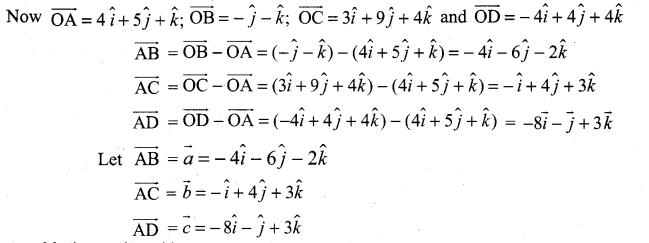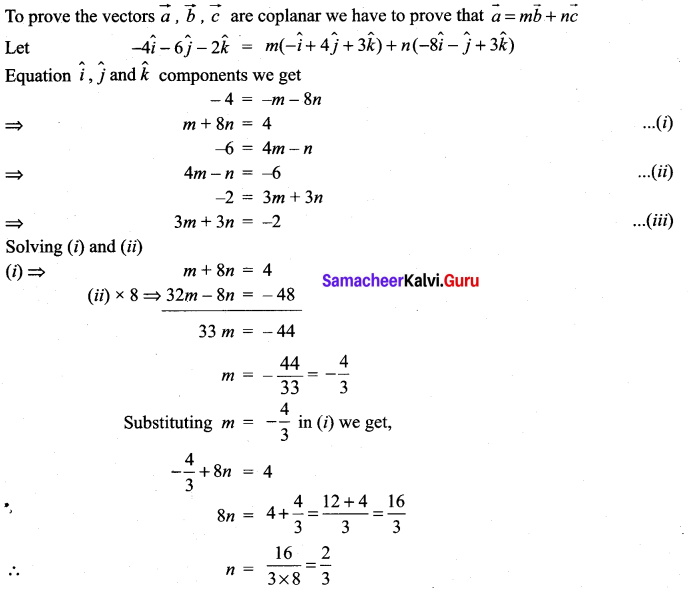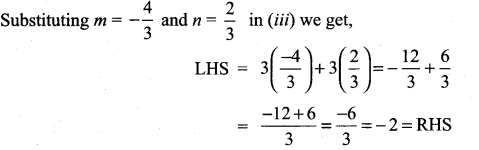∴ we are able to write one vector as a linear combination of the other two vectors ⇒ the given vectors $$\vec{a}$$, $$\vec{b}$$, $$\vec{c}$$ are coplanar.
(i.e.,) the given points A, B, C, D are coplanar.

Question 11.
If $$\vec{a}=2 \hat{i}+3 \hat{j}-4 \hat{k}$$, $$\vec{b}=3 \hat{i}-4 \hat{j}-5 \hat{k}$$ and $$\vec{c}=-3 \hat{i}+2 \hat{j}+3 \hat{k}$$, find the magnitude and direction cosines of
(i) $$\vec{a}+\vec{b}+\vec{c}$$
(ii) $$3 \vec{a}-2 \vec{b}+5 \vec{c}$$
Solution: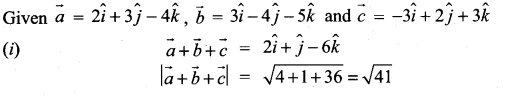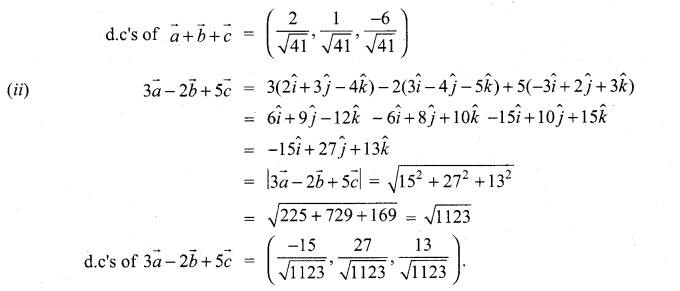>

Question 12.
The position vectors of the vertices of a triangle are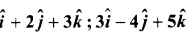and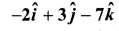. Find the perimeter of the triangle
Solution:
Let A, B, C be the vertices of the triangle ABC,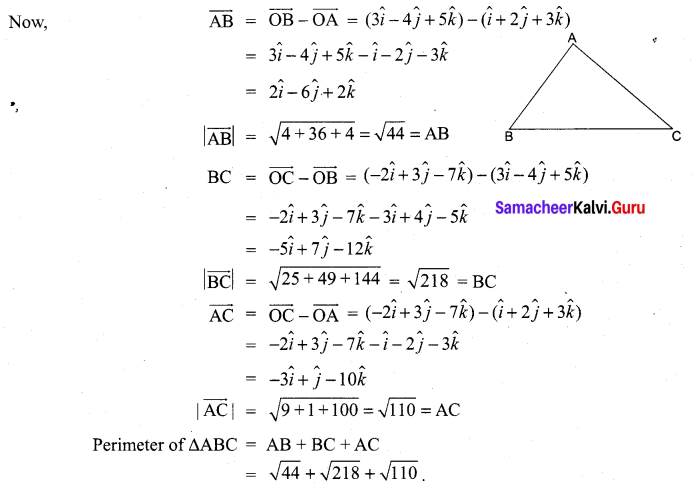Question 13.
Find the unit vector parallel to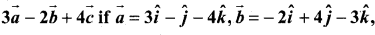and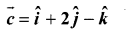Solution: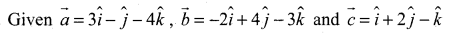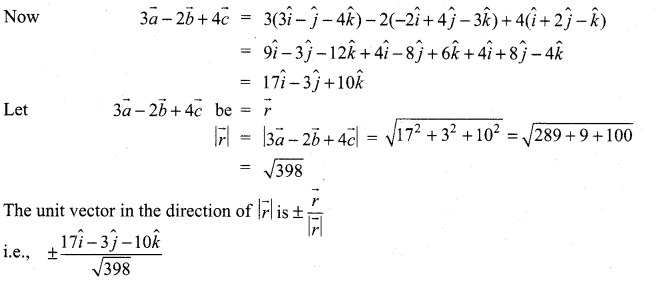Question 14.
The position vector $$\vec{a}$$, $$\vec{b}$$, $$\vec{c}$$ three points satisfy the relation $$2 \vec{a}-7 \vec{b}+5 \vec{c}=\overrightarrow{0}$$. Are these points collinear?
Solution: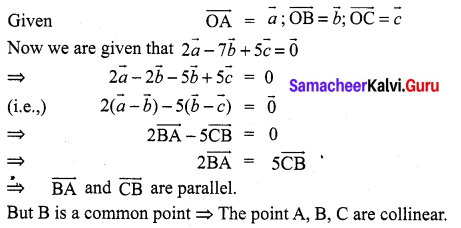Question 15.
The position vectors of the point P, Q, R, S are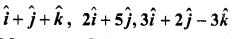and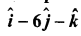respectively. Prove that the line PQ and RS are parallel.
Solution: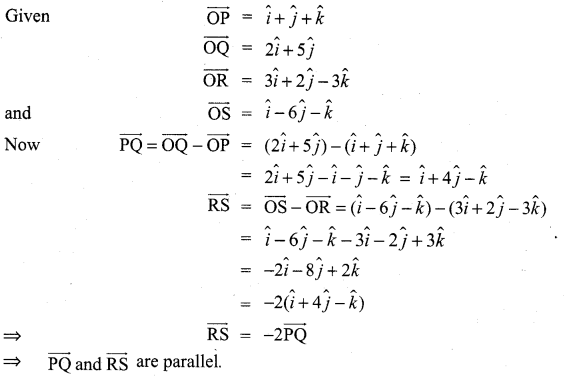Question 16.
Find the value or values of m for which $$m(\hat{i}+\hat{j}+\hat{k})$$ is a unit vector
Solution: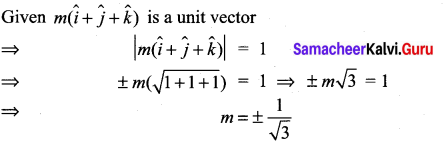Question 17.
Show that the points A(1, 1, 1), B(1, 2, 3) and C(2, -1, 1) are vertices of an isosceles triangle.
Solution: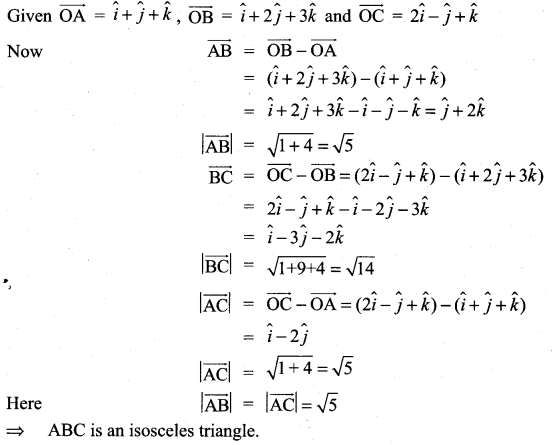### Samacheer Kalvi 11th Maths Solutions Chapter 8 Vector Algebra – I Ex 8.2 Additional Problems

Question 1.
Show that the points whose position vectors given by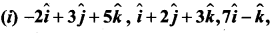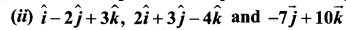Solution: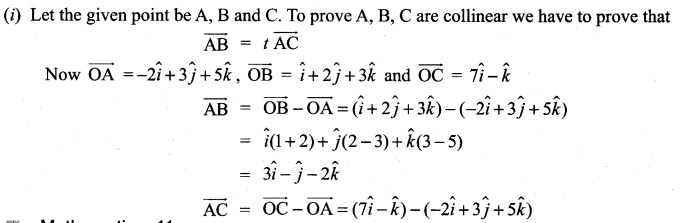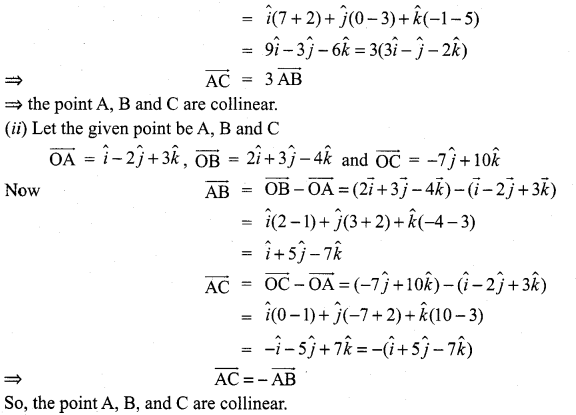Question 2.
Find the unit vectors parallel to the sum of $$3 \hat{i}-5 \hat{j}+8 \hat{k}$$ and $$-2 \hat{j}-2 \hat{k}$$
Solution: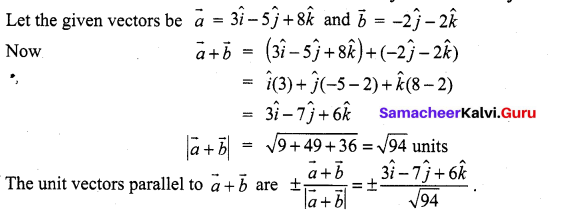Question 3.
The vertices of a triangle have position vectors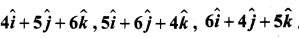Prove that the triangle is equilateral.
Solution: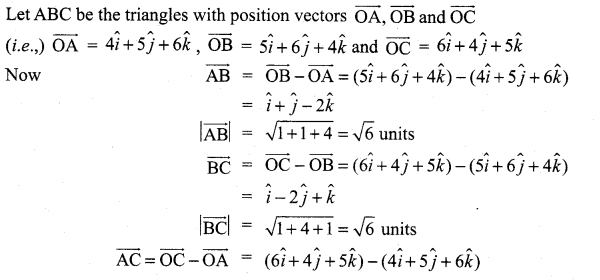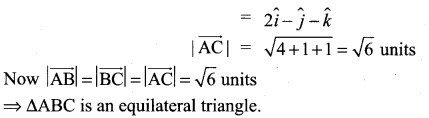Question 4.
Prove that the points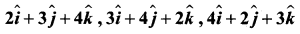form an equilateral triangle.
Solution: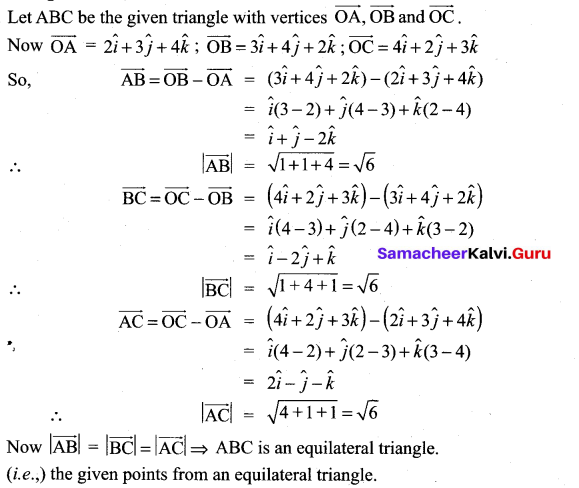Question 5.
Examine whether the vectors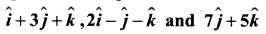are coplanar
Solution: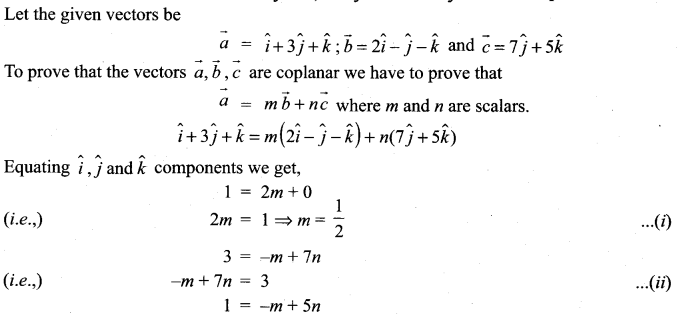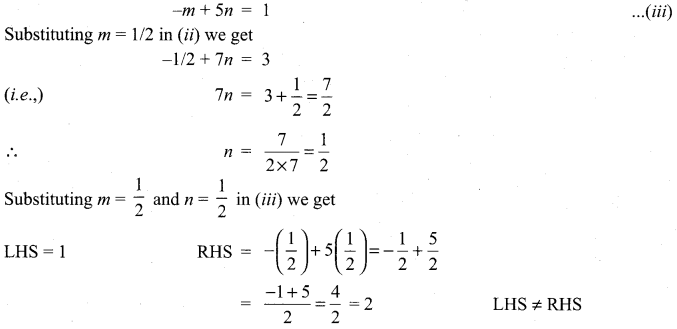⇒ We are not able to write one vector as a linear combination of the other two vectors
⇒ the given vectors are not coplanar.